Theory and Modern Applications

# Existence results for nonlinear fractional differential equations with closed boundary conditions and impulses

## Abstract

This paper is concerned with the existence and uniqueness of solutions for impulsive nonlinear differential equations of fractional order $\alpha \in \left(1,2\right]$ with closed boundary conditions. By applying some standard fixed point theorems, we obtain the sufficient conditions for the existence and uniqueness of solutions of the problem at hand. An illustrative example is presented.

MSC:26A33, 34B15, 34B37.

## 1 Introduction

Dynamical systems with impulse effect are regarded as a class of general hybrid systems. Impulsive hybrid systems are composed of some continuous variable dynamic systems along with certain reset maps that define impulsive switching among them. It is the switching that resets the modes and changes the continuous state of the system. There are three classes of impulsive hybrid systems, namely impulsive differential systems [1, 2], sampled data or digital control system [3, 4], and impulsive switched system . Using hybrid models, one may represent time and event-based behaviors more accurately so as to meet challenging design requirements in the design of control systems for problems such as cut-off control and idle speed control of the engine. For more details, see  and the references therein.

Fractional calculus (differentiation and integration of arbitrary order) has proved to be an important tool in the modeling of dynamical systems associated with phenomena such as fractals and chaos. In fact, this branch of calculus has found its applications in various disciplines of science and engineering such as mechanics, electricity, chemistry, biology, economics, control theory, signal and image processing, polymer rheology, regular variation in thermodynamics, biophysics, blood flow phenomena, aerodynamics, electro-dynamics of complex medium, viscoelasticity and damping, control theory, wave propagation, percolation, identification, fitting of experimental data, etc. Fractional derivatives provide an excellent tool for the description of memory and hereditary properties of various materials and processes. With this advantage, the fractional-order models become more realistic and practical than the classical integer-order models in which such effects are not taken into account. For some recent details and examples, see  and the references therein.

Impulsive differential equations are found to be important mathematical tools for better understanding of several real world problems in biology, physics, engineering, etc. In fact, the theory of impulsive differential equations of integer order has found its extensive applications in realistic mathematical modeling of a wide variety of practical situations and has emerged as an important area of investigation; for instance, see  and references therein. The recent surge in developing the theory of differential equations of fractional order has led several researchers to study the fractional differential equations with impulse effects. For some recent work on impulsive differential equations of fractional order, see  and the references therein.

In this paper, we investigate the existence of solutions for the following impulsive fractional differential equations with closed boundary conditions:

$\left\{\begin{array}{c}{}^{C}D^{q}x\left(t\right)=f\left(t,x\left(t\right)\right),\phantom{\rule{1em}{0ex}}1
(1.1)

where ${}^{C}D^{q}$ is the Caputo fractional derivative, $f\in C\left(J×\mathbb{R},\mathbb{R}\right)$, ${I}_{k},{I}_{k}^{\ast }\in C\left(\mathbb{R},\mathbb{R}\right)$, $J=\left[0,T\right]$ ($T>0$), $0={t}_{0}<{t}_{1}<\cdots <{t}_{k}<\cdots <{t}_{p}<{t}_{p+1}=T$, ${J}^{\prime }=J\mathrm{\setminus }\left\{{t}_{1},{t}_{2},\dots ,{t}_{p}\right\}$, $\mathrm{△}x\left({t}_{k}\right)=x\left({t}_{k}^{+}\right)-x\left({t}_{k}^{-}\right)$, where $x\left({t}_{k}^{+}\right)$ and $x\left({t}_{k}^{-}\right)$ denote the right and the left limits of $x\left(t\right)$ at $t={t}_{k}\left(k=1,2,\dots ,p\right)$, respectively. $\mathrm{△}{x}^{\prime }\left({t}_{k}\right)$ have a similar meaning for ${x}^{\prime }\left(t\right)$.

Here we remark that the boundary conditions in (1.1) include quasi-periodic boundary conditions ($\beta =\gamma =0$) and interpolate between periodic ($\alpha ,\delta \to 1$, $\beta ,\gamma \to 0$) and antiperiodic ($\alpha =\delta =-1$, $\beta =\gamma =0$) boundary conditions. For more details and applications of closed boundary conditions, see .

## 2 Preliminaries

Let ${J}_{0}=\left[0,{t}_{1}\right],{J}_{1}=\left({t}_{1},{t}_{2}\right],\dots ,{J}_{p-1}=\left({t}_{p-1},{t}_{p}\right],{J}_{p}=\left({t}_{p},T\right]$, and we introduce the spaces: with the norm $\parallel x\parallel ={sup}_{t\in J}|x\left(t\right)|$, and with the norm ${\parallel x\parallel }_{P{C}^{1}}=max\left\{\parallel x\parallel ,\parallel {x}^{\prime }\parallel \right\}$. Obviously, $PC\left(J,\mathbb{R}\right)$ and $P{C}^{1}\left(J,\mathbb{R}\right)$ are Banach spaces.

In passing, we remark that ${}^{C}D^{q}x\left(t\right)$ indeed stands for ${}^{C}D_{{t}_{k}}^{q}x\left(t\right)$ for t in the subinterval $\left({t}_{k},{t}_{k+1}\right]$.

Definition 2.1 A function $x\in P{C}^{1}\left(J,\mathbb{R}\right)$ with its Caputo derivative of order q existing on J is a solution of (1.1) if it satisfies (1.1).

Define

${\lambda }_{1}\left(t\right)=\frac{\left(1-\delta \right)T+\gamma t}{T\mathrm{\Lambda }},\phantom{\rule{2em}{0ex}}{\lambda }_{2}\left(t\right)=\frac{\left(1-\beta \right)T-\left(1-\alpha \right)t}{\mathrm{\Lambda }},$

where $\mathrm{\Lambda }=\left(1-\alpha \right)\left(1-\delta \right)+\gamma \left(1-\beta \right)\ne 0$.

Lemma 2.1 For a given $y\in C\left[0,T\right]$, a function x is a solution of the impulsive closed boundary value problem

$\left\{\begin{array}{c}{}^{C}D^{q}x\left(t\right)=y\left(t\right),\phantom{\rule{1em}{0ex}}1<\alpha \le 2,t\in {J}^{\prime },\hfill \\ \mathrm{△}x\left({t}_{k}\right)={I}_{k}\left(x\left({t}_{k}\right)\right),\phantom{\rule{2em}{0ex}}\mathrm{△}{x}^{\prime }\left({t}_{k}\right)={I}_{k}^{\ast }\left(x\left({t}_{k}\right)\right),\phantom{\rule{1em}{0ex}}k=1,2,\dots ,p,\hfill \\ x\left(T\right)=\alpha x\left(0\right)+\beta T{x}^{\prime }\left(0\right),\phantom{\rule{2em}{0ex}}T{x}^{\prime }\left(T\right)=\gamma x\left(0\right)+\delta T{x}^{\prime }\left(0\right),\hfill \end{array}$
(2.1)

if and only if x is a solution of the impulsive fractional integral equation

$x\left(t\right)=\left\{\begin{array}{c}{\int }_{0}^{t}\frac{{\left(t-s\right)}^{q-1}}{\mathrm{\Gamma }\left(q\right)}y\left(s\right)\phantom{\rule{0.2em}{0ex}}ds-{\lambda }_{1}\left(t\right){\int }_{{t}_{p}}^{T}\frac{{\left(T-s\right)}^{q-1}}{\mathrm{\Gamma }\left(q\right)}y\left(s\right)\phantom{\rule{0.2em}{0ex}}ds\hfill \\ \phantom{\rule{1em}{0ex}}+{\lambda }_{2}\left(t\right){\int }_{{t}_{p}}^{T}\frac{{\left(T-s\right)}^{q-2}}{\mathrm{\Gamma }\left(q-1\right)}y\left(s\right)\phantom{\rule{0.2em}{0ex}}ds+\mathcal{A},\phantom{\rule{1em}{0ex}}t\in {J}_{0};\hfill \\ {\int }_{{t}_{k}}^{t}\frac{{\left(t-s\right)}^{q-1}}{\mathrm{\Gamma }\left(q\right)}y\left(s\right)\phantom{\rule{0.2em}{0ex}}ds-{\lambda }_{1}\left(t\right){\int }_{{t}_{p}}^{T}\frac{{\left(T-s\right)}^{q-1}}{\mathrm{\Gamma }\left(q\right)}y\left(s\right)\phantom{\rule{0.2em}{0ex}}ds\hfill \\ \phantom{\rule{1em}{0ex}}+{\lambda }_{2}\left(t\right){\int }_{{t}_{p}}^{T}\frac{{\left(T-s\right)}^{q-2}}{\mathrm{\Gamma }\left(q-1\right)}y\left(s\right)\phantom{\rule{0.2em}{0ex}}ds\hfill \\ \phantom{\rule{1em}{0ex}}+{\sum }_{i=1}^{k}\left[{\int }_{{t}_{i-1}}^{{t}_{i}}\frac{{\left({t}_{i}-s\right)}^{q-1}}{\mathrm{\Gamma }\left(q\right)}y\left(s\right)\phantom{\rule{0.2em}{0ex}}ds+{I}_{i}\left(x\left({t}_{i}\right)\right)\right]\hfill \\ \phantom{\rule{1em}{0ex}}+{\sum }_{i=1}^{k-1}\left({t}_{k}-{t}_{i}\right)\left[{\int }_{{t}_{i-1}}^{{t}_{i}}\frac{{\left({t}_{i}-s\right)}^{q-2}}{\mathrm{\Gamma }\left(q-1\right)}y\left(s\right)\phantom{\rule{0.2em}{0ex}}ds+{I}_{i}^{\ast }\left(x\left({t}_{i}\right)\right)\right]\hfill \\ \phantom{\rule{1em}{0ex}}+{\sum }_{i=1}^{k}\left(t-{t}_{k}\right)\left[{\int }_{{t}_{i-1}}^{{t}_{i}}\frac{{\left({t}_{i}-s\right)}^{q-2}}{\mathrm{\Gamma }\left(q-1\right)}y\left(s\right)\phantom{\rule{0.2em}{0ex}}ds+{I}_{i}^{\ast }\left(x\left({t}_{i}\right)\right)\right]\hfill \\ \phantom{\rule{1em}{0ex}}+\mathcal{A},\phantom{\rule{1em}{0ex}}t\in {J}_{k},k=1,2,\dots ,p,\hfill \end{array}$
(2.2)

where

$\begin{array}{rcl}\mathcal{A}& =& -{\lambda }_{1}\left(t\right)\sum _{i=1}^{p}\left[{\int }_{{t}_{i-1}}^{{t}_{i}}\frac{{\left({t}_{i}-s\right)}^{q-1}}{\mathrm{\Gamma }\left(q\right)}y\left(s\right)\phantom{\rule{0.2em}{0ex}}ds+{I}_{i}\left(x\left({t}_{i}\right)\right)\right]\\ -{\lambda }_{1}\left(t\right)\sum _{i=1}^{p-1}\left({t}_{p}-{t}_{i}\right)\left[{\int }_{{t}_{i-1}}^{{t}_{i}}\frac{{\left({t}_{i}-s\right)}^{q-2}}{\mathrm{\Gamma }\left(q-1\right)}y\left(s\right)\phantom{\rule{0.2em}{0ex}}ds+{I}_{i}^{\ast }\left(x\left({t}_{i}\right)\right)\right]\\ -\sum _{i=1}^{p}\left[\left(T-{t}_{p}\right){\lambda }_{1}\left(t\right)-{\lambda }_{2}\left(t\right)\right]\left[{\int }_{{t}_{i-1}}^{{t}_{i}}\frac{{\left({t}_{i}-s\right)}^{q-2}}{\mathrm{\Gamma }\left(q-1\right)}y\left(s\right)\phantom{\rule{0.2em}{0ex}}ds+{I}_{i}^{\ast }\left(x\left({t}_{i}\right)\right)\right].\end{array}$

Proof Let x be a solution of (2.1). Then, for $t\in {J}_{0}$, there exist constants ${c}_{1},{c}_{2}\in \mathbb{R}$ such that

$\begin{array}{r}x\left(t\right)={\mathcal{I}}^{q}y\left(t\right)-{c}_{1}-{c}_{2}t=\frac{1}{\mathrm{\Gamma }\left(q\right)}{\int }_{0}^{t}{\left(t-s\right)}^{q-1}y\left(s\right)\phantom{\rule{0.2em}{0ex}}ds-{c}_{1}-{c}_{2}t,\\ {x}^{\prime }\left(t\right)=\frac{1}{\mathrm{\Gamma }\left(q-1\right)}{\int }_{0}^{t}{\left(t-s\right)}^{q-2}y\left(s\right)\phantom{\rule{0.2em}{0ex}}ds-{c}_{2}.\end{array}$
(2.3)

For $t\in {J}_{1}$, there exist constants ${d}_{1},{d}_{2}\in \mathbb{R}$, such that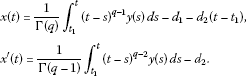Then we have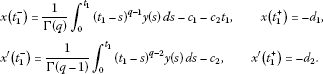In view of the impulse conditions $\mathrm{△}x\left({t}_{1}\right)=x\left({t}_{1}^{+}\right)-x\left({t}_{1}^{-}\right)={I}_{1}\left(x\left({t}_{1}\right)\right)$ and $\mathrm{△}{x}^{\prime }\left({t}_{1}\right)={x}^{\prime }\left({t}_{1}^{+}\right)-{x}^{\prime }\left({t}_{1}^{-}\right)={I}_{1}^{\ast }\left(x\left({t}_{1}\right)\right)$, we have that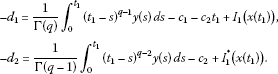Consequently,

$\begin{array}{rcl}x\left(t\right)& =& \frac{1}{\mathrm{\Gamma }\left(q\right)}{\int }_{{t}_{1}}^{t}{\left(t-s\right)}^{q-1}y\left(s\right)\phantom{\rule{0.2em}{0ex}}ds+\frac{1}{\mathrm{\Gamma }\left(q\right)}{\int }_{0}^{{t}_{1}}{\left({t}_{1}-s\right)}^{q-1}y\left(s\right)\phantom{\rule{0.2em}{0ex}}ds\\ +\frac{t-{t}_{1}}{\mathrm{\Gamma }\left(q-1\right)}{\int }_{0}^{{t}_{1}}{\left({t}_{1}-s\right)}^{q-2}y\left(s\right)\phantom{\rule{0.2em}{0ex}}ds+{I}_{1}\left(x\left({t}_{1}\right)\right)+\left(t-{t}_{1}\right){I}_{1}^{\ast }\left(x\left({t}_{1}\right)\right)-{c}_{1}-{c}_{2}t,\phantom{\rule{1em}{0ex}}t\in {J}_{1}.\end{array}$

By a similar process, we can get

$\begin{array}{rcl}x\left(t\right)& =& {\int }_{{t}_{k}}^{t}\frac{{\left(t-s\right)}^{q-1}}{\mathrm{\Gamma }\left(q\right)}y\left(s\right)\phantom{\rule{0.2em}{0ex}}ds+\sum _{i=1}^{k}\left[{\int }_{{t}_{i-1}}^{{t}_{i}}\frac{{\left({t}_{i}-s\right)}^{q-1}}{\mathrm{\Gamma }\left(q\right)}y\left(s\right)\phantom{\rule{0.2em}{0ex}}ds+{I}_{i}\left(x\left({t}_{i}\right)\right)\right]\\ +\sum _{i=1}^{k-1}\left({t}_{k}-{t}_{i}\right)\left[{\int }_{{t}_{i-1}}^{{t}_{i}}\frac{{\left({t}_{i}-s\right)}^{q-2}}{\mathrm{\Gamma }\left(q-1\right)}y\left(s\right)\phantom{\rule{0.2em}{0ex}}ds+{I}_{i}^{\ast }\left(x\left({t}_{i}\right)\right)\right]\\ +\sum _{i=1}^{k}\left(t-{t}_{k}\right)\left[{\int }_{{t}_{i-1}}^{{t}_{i}}\frac{{\left({t}_{i}-s\right)}^{q-2}}{\mathrm{\Gamma }\left(q-1\right)}y\left(s\right)\phantom{\rule{0.2em}{0ex}}ds+{I}_{i}^{\ast }\left(x\left({t}_{i}\right)\right)\right]\\ -{c}_{1}-{c}_{2}t,\phantom{\rule{1em}{0ex}}t\in {J}_{k},k=1,2,\dots ,p.\end{array}$
(2.4)

Using the conditions $x\left(T\right)=\alpha x\left(0\right)+\beta T{x}^{\prime }\left(0\right)$ and $T{x}^{\prime }\left(T\right)=\gamma x\left(0\right)+\delta T{x}^{\prime }\left(0\right)$, we find that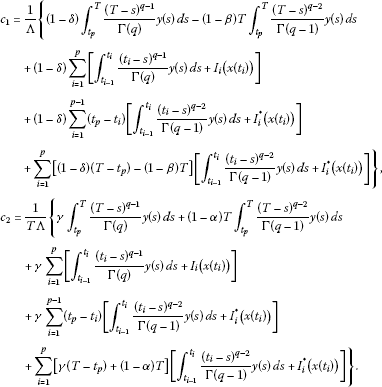Substituting the value of ${c}_{1}$, ${c}_{2}$ in (2.3) and (2.4), we obtain (2.2). Conversely, assume that u is a solution of the impulsive fractional integral equation (2.2), then by a direct computation, it follows that the solution given by (2.2) satisfies (2.1). □

## 3 Main results

Define an operator $\mathfrak{G}:PC\left(J,\mathbb{R}\right)\to PC\left(J,\mathbb{R}\right)$ as

$\begin{array}{rcl}\mathfrak{G}x\left(t\right)& =& {\int }_{{t}_{k}}^{t}\frac{{\left(t-s\right)}^{q-1}}{\mathrm{\Gamma }\left(q\right)}f\left(s,x\left(s\right)\right)\phantom{\rule{0.2em}{0ex}}ds-{\lambda }_{1}\left(t\right){\int }_{{t}_{p}}^{T}\frac{{\left(T-s\right)}^{q-1}}{\mathrm{\Gamma }\left(q\right)}f\left(s,x\left(s\right)\right)\phantom{\rule{0.2em}{0ex}}ds\\ +{\lambda }_{2}\left(t\right){\int }_{{t}_{p}}^{T}\frac{{\left(T-s\right)}^{q-2}}{\mathrm{\Gamma }\left(q-1\right)}f\left(s,x\left(s\right)\right)\phantom{\rule{0.2em}{0ex}}ds+\sum _{i=1}^{k}\left[{\int }_{{t}_{i-1}}^{{t}_{i}}\frac{{\left({t}_{i}-s\right)}^{q-1}}{\mathrm{\Gamma }\left(q\right)}f\left(s,x\left(s\right)\right)\phantom{\rule{0.2em}{0ex}}ds+{I}_{i}\left(x\left({t}_{i}\right)\right)\right]\\ +\sum _{i=1}^{k-1}\left({t}_{k}-{t}_{i}\right)\left[{\int }_{{t}_{i-1}}^{{t}_{i}}\frac{{\left({t}_{i}-s\right)}^{q-2}}{\mathrm{\Gamma }\left(q-1\right)}f\left(s,x\left(s\right)\right)\phantom{\rule{0.2em}{0ex}}ds+{I}_{i}^{\ast }\left(x\left({t}_{i}\right)\right)\right]\\ +\sum _{i=1}^{k}\left(t-{t}_{k}\right)\left[{\int }_{{t}_{i-1}}^{{t}_{i}}\frac{{\left({t}_{i}-s\right)}^{q-2}}{\mathrm{\Gamma }\left(q-1\right)}f\left(s,x\left(s\right)\right)\phantom{\rule{0.2em}{0ex}}ds+{I}_{i}^{\ast }\left(x\left({t}_{i}\right)\right)\right]\\ -{\lambda }_{1}\left(t\right)\sum _{i=1}^{p}\left[{\int }_{{t}_{i-1}}^{{t}_{i}}\frac{{\left({t}_{i}-s\right)}^{q-1}}{\mathrm{\Gamma }\left(q\right)}f\left(s,x\left(s\right)\right)\phantom{\rule{0.2em}{0ex}}ds+{I}_{i}\left(x\left({t}_{i}\right)\right)\right]\\ -{\lambda }_{1}\left(t\right)\sum _{i=1}^{p-1}\left({t}_{p}-{t}_{i}\right)\left[{\int }_{{t}_{i-1}}^{{t}_{i}}\frac{{\left({t}_{i}-s\right)}^{q-2}}{\mathrm{\Gamma }\left(q-1\right)}f\left(s,x\left(s\right)\right)\phantom{\rule{0.2em}{0ex}}ds+{I}_{i}^{\ast }\left(x\left({t}_{i}\right)\right)\right]\\ -\sum _{i=1}^{p}\left[\left(T-{t}_{p}\right){\lambda }_{1}\left(t\right)-{\lambda }_{2}\left(t\right)\right]\left[{\int }_{{t}_{i-1}}^{{t}_{i}}\frac{{\left({t}_{i}-s\right)}^{q-2}}{\mathrm{\Gamma }\left(q-1\right)}f\left(s,x\left(s\right)\right)\phantom{\rule{0.2em}{0ex}}ds+{I}_{i}^{\ast }\left(x\left({t}_{i}\right)\right)\right].\end{array}$
(3.1)

Observe that the problem (1.1) has a solution if and only if the operator T has a fixed point.

Lemma 3.1 The operator $\mathfrak{G}:PC\left(J,\mathbb{R}\right)\to PC\left(J,\mathbb{R}\right)$ defined by (3.1) is completely continuous.

Proof It is obvious that $\mathfrak{G}$ is continuous in view of continuity of f, ${I}_{k}$ and ${I}_{k}^{\ast }$.

Let $\mathrm{\Omega }\subset PC\left(J,\mathbb{R}\right)$ be bounded. Then, there exist positive constants ${L}_{i}>0$ ($i=1,2,3$) such that $|f\left(t,x\right)|\le {L}_{1}$, $|{I}_{k}\left(x\right)|\le {L}_{2}$ and $|{I}_{k}^{\ast }\left(x\right)|\le {L}_{3}$, $\mathrm{\forall }x\in \mathrm{\Omega }$. Thus, $\mathrm{\forall }x\in \mathrm{\Omega }$, we have

$\begin{array}{rcl}|\mathfrak{G}x\left(t\right)|& \le & {\int }_{{t}_{k}}^{t}\frac{{\left(t-s\right)}^{q-1}}{\mathrm{\Gamma }\left(q\right)}|f\left(s,x\left(s\right)\right)|\phantom{\rule{0.2em}{0ex}}ds+|{\lambda }_{1}\left(t\right)|{\int }_{{t}_{p}}^{T}\frac{{\left(T-s\right)}^{q-1}}{\mathrm{\Gamma }\left(q\right)}|f\left(s,x\left(s\right)\right)|\phantom{\rule{0.2em}{0ex}}ds\\ +|{\lambda }_{2}\left(t\right)|{\int }_{{t}_{p}}^{T}\frac{{\left(T-s\right)}^{q-2}}{\mathrm{\Gamma }\left(q-1\right)}|f\left(s,x\left(s\right)\right)|\phantom{\rule{0.2em}{0ex}}ds\\ +\sum _{i=1}^{k}\left[{\int }_{{t}_{i-1}}^{{t}_{i}}\frac{{\left({t}_{i}-s\right)}^{q-1}}{\mathrm{\Gamma }\left(q\right)}|f\left(s,x\left(s\right)\right)|\phantom{\rule{0.2em}{0ex}}ds+|{I}_{i}\left(x\left({t}_{i}\right)\right)|\right]\\ +\sum _{i=1}^{k-1}\left({t}_{k}-{t}_{i}\right)\left[{\int }_{{t}_{i-1}}^{{t}_{i}}\frac{{\left({t}_{i}-s\right)}^{q-2}}{\mathrm{\Gamma }\left(q-1\right)}|f\left(s,x\left(s\right)\right)|\phantom{\rule{0.2em}{0ex}}ds+|{I}_{i}^{\ast }\left(x\left({t}_{i}\right)\right)|\right]\\ +\sum _{i=1}^{k}\left(t-{t}_{k}\right)\left[{\int }_{{t}_{i-1}}^{{t}_{i}}\frac{{\left({t}_{i}-s\right)}^{q-2}}{\mathrm{\Gamma }\left(q-1\right)}|f\left(s,x\left(s\right)\right)|\phantom{\rule{0.2em}{0ex}}ds+|{I}_{i}^{\ast }\left(x\left({t}_{i}\right)\right)|\right]\\ +|{\lambda }_{1}\left(t\right)|\sum _{i=1}^{p}\left[{\int }_{{t}_{i-1}}^{{t}_{i}}\frac{{\left({t}_{i}-s\right)}^{q-1}}{\mathrm{\Gamma }\left(q\right)}|f\left(s,x\left(s\right)\right)|\phantom{\rule{0.2em}{0ex}}ds+|{I}_{i}\left(x\left({t}_{i}\right)\right)|\right]\\ +|{\lambda }_{1}\left(t\right)|\sum _{i=1}^{p-1}\left({t}_{p}-{t}_{i}\right)\left[{\int }_{{t}_{i-1}}^{{t}_{i}}\frac{{\left({t}_{i}-s\right)}^{q-2}}{\mathrm{\Gamma }\left(q-1\right)}|f\left(s,x\left(s\right)\right)|\phantom{\rule{0.2em}{0ex}}ds+|{I}_{i}^{\ast }\left(x\left({t}_{i}\right)\right)|\right]\\ +\sum _{i=1}^{p}\left[\left(T-{t}_{p}\right)|{\lambda }_{1}\left(t\right)|+|{\lambda }_{2}\left(t\right)|\right]\left[{\int }_{{t}_{i-1}}^{{t}_{i}}\frac{{\left({t}_{i}-s\right)}^{q-2}}{\mathrm{\Gamma }\left(q-1\right)}|f\left(s,x\left(s\right)\right)|\phantom{\rule{0.2em}{0ex}}ds+|{I}_{i}^{\ast }\left(x\left({t}_{i}\right)\right)|\right]\\ \le & {L}_{1}{\int }_{{t}_{k}}^{t}\frac{{\left(t-s\right)}^{q-1}}{\mathrm{\Gamma }\left(q\right)}\phantom{\rule{0.2em}{0ex}}ds+|{\lambda }_{1}\left(t\right)|{L}_{1}{\int }_{{t}_{p}}^{T}\frac{{\left(T-s\right)}^{q-1}}{\mathrm{\Gamma }\left(q\right)}\phantom{\rule{0.2em}{0ex}}ds+|{\lambda }_{2}\left(t\right)|{L}_{1}{\int }_{{t}_{p}}^{T}\frac{{\left(T-s\right)}^{q-2}}{\mathrm{\Gamma }\left(q-1\right)}\phantom{\rule{0.2em}{0ex}}ds\\ +\sum _{i=1}^{p}\left[{L}_{1}{\int }_{{t}_{i-1}}^{{t}_{i}}\frac{{\left({t}_{i}-s\right)}^{q-1}}{\mathrm{\Gamma }\left(q\right)}\phantom{\rule{0.2em}{0ex}}ds+{L}_{2}\right]+\sum _{i=1}^{p-1}T\left[{L}_{1}{\int }_{{t}_{i-1}}^{{t}_{i}}\frac{{\left({t}_{i}-s\right)}^{q-2}}{\mathrm{\Gamma }\left(q-1\right)}\phantom{\rule{0.2em}{0ex}}ds+{L}_{3}\right]\\ +\sum _{i=1}^{p}T\left[{L}_{1}{\int }_{{t}_{i-1}}^{{t}_{i}}\frac{{\left({t}_{i}-s\right)}^{q-2}}{\mathrm{\Gamma }\left(q-1\right)}\phantom{\rule{0.2em}{0ex}}ds+{L}_{3}\right]+|{\lambda }_{1}\left(t\right)|\sum _{i=1}^{p}\left[{L}_{1}{\int }_{{t}_{i-1}}^{{t}_{i}}\frac{{\left({t}_{i}-s\right)}^{q-1}}{\mathrm{\Gamma }\left(q\right)}\phantom{\rule{0.2em}{0ex}}ds+{L}_{2}\right]\\ +|{\lambda }_{1}\left(t\right)|\sum _{i=1}^{p-1}T\left[{L}_{1}{\int }_{{t}_{i-1}}^{{t}_{i}}\frac{{\left({t}_{i}-s\right)}^{q-2}}{\mathrm{\Gamma }\left(q-1\right)}\phantom{\rule{0.2em}{0ex}}ds+{L}_{3}\right]\\ +\sum _{i=1}^{p}\left[T|{\lambda }_{1}\left(t\right)|+|{\lambda }_{2}\left(t\right)|\right]\left[{L}_{1}{\int }_{{t}_{i-1}}^{{t}_{i}}\frac{{\left({t}_{i}-s\right)}^{q-2}}{\mathrm{\Gamma }\left(q-1\right)}\phantom{\rule{0.2em}{0ex}}ds+{L}_{3}\right]\\ \le & \frac{\left[1+p+q\left(2p-1\right)\right]\left(1+|{\lambda }_{1}\left(t\right)|\right){T}^{q}{L}_{1}}{\mathrm{\Gamma }\left(q+1\right)}+\frac{\left(1+p\right)|{\lambda }_{2}\left(t\right)|{T}^{q-1}{L}_{1}}{\mathrm{\Gamma }\left(q\right)}+p\left(1+|{\lambda }_{1}\left(t\right)|\right){L}_{2}\\ +\left[\left(2p-1\right)T\left(1+|{\lambda }_{1}\left(t\right)|\right)+p|{\lambda }_{2}\left(t\right)|\right]{L}_{3}\\ \le & \underset{t\in J}{max}\left\{\frac{\left[1+p+q\left(2p-1\right)\right]\left(1+|{\lambda }_{1}\left(t\right)|\right){T}^{q}{L}_{1}}{\mathrm{\Gamma }\left(q+1\right)}\\ +\frac{\left(1+p\right)|{\lambda }_{2}\left(t\right)|{T}^{q-1}{L}_{1}}{\mathrm{\Gamma }\left(q\right)}+p\left(1+|{\lambda }_{1}\left(t\right)|\right){L}_{2}\\ +\left[\left(2p-1\right)T\left(1+|{\lambda }_{1}\left(t\right)|\right)+p|{\lambda }_{2}\left(t\right)|\right]{L}_{3}\right\}:=L,\end{array}$
(3.2)

which implies that $\parallel \mathfrak{G}x\parallel \le L$.

On the other hand, for any $t\in {J}_{k}$, $0\le k\le p$, we have

$\begin{array}{rcl}|{\left(\mathfrak{G}x\right)}^{\prime }\left(t\right)|& \le & {\int }_{{t}_{k}}^{t}\frac{{\left(t-s\right)}^{q-2}}{\mathrm{\Gamma }\left(q-1\right)}|f\left(s,x\left(s\right)\right)|\phantom{\rule{0.2em}{0ex}}ds+\frac{|\gamma |}{T|\mathrm{\Lambda }|}{\int }_{{t}_{p}}^{T}\frac{{\left(T-s\right)}^{q-1}}{\mathrm{\Gamma }\left(q\right)}|f\left(s,x\left(s\right)\right)|\phantom{\rule{0.2em}{0ex}}ds\\ +\frac{|1-\alpha |}{|\mathrm{\Lambda }|}{\int }_{{t}_{p}}^{T}\frac{{\left(T-s\right)}^{q-2}}{\mathrm{\Gamma }\left(q-1\right)}|f\left(s,x\left(s\right)\right)|\phantom{\rule{0.2em}{0ex}}ds\\ +\sum _{i=1}^{p}\left[{\int }_{{t}_{i-1}}^{{t}_{i}}\frac{{\left({t}_{i}-s\right)}^{q-2}}{\mathrm{\Gamma }\left(q-1\right)}|f\left(s,x\left(s\right)\right)|\phantom{\rule{0.2em}{0ex}}ds+|{I}_{i}^{\ast }\left(u\left({t}_{i}\right)\right)|\right]\\ +\frac{|\gamma |}{T|\mathrm{\Lambda }|}\sum _{i=1}^{p}\left[{\int }_{{t}_{i-1}}^{{t}_{i}}\frac{{\left({t}_{i}-s\right)}^{q-1}}{\mathrm{\Gamma }\left(q\right)}|f\left(s,x\left(s\right)\right)|\phantom{\rule{0.2em}{0ex}}ds+|{I}_{i}\left(u\left({t}_{i}\right)\right)|\right]\\ +\frac{|\gamma |}{T|\mathrm{\Lambda }|}\sum _{i=1}^{p-1}\left({t}_{p}-{t}_{i}\right)\left[{\int }_{{t}_{i-1}}^{{t}_{i}}\frac{{\left({t}_{i}-s\right)}^{q-2}}{\mathrm{\Gamma }\left(q-1\right)}|f\left(s,x\left(s\right)\right)|\phantom{\rule{0.2em}{0ex}}ds+|{I}_{i}^{\ast }\left(u\left({t}_{i}\right)\right)|\right]\\ +\sum _{i=1}^{p}\frac{|\gamma |+|1-\alpha |}{|\mathrm{\Lambda }|}\left[{\int }_{{t}_{i-1}}^{{t}_{i}}\frac{{\left({t}_{i}-s\right)}^{q-2}}{\mathrm{\Gamma }\left(q-1\right)}|f\left(s,x\left(s\right)\right)|\phantom{\rule{0.2em}{0ex}}ds+|{I}_{i}^{\ast }\left(u\left({t}_{i}\right)\right)|\right]\\ \le & {L}_{1}{\int }_{{t}_{k}}^{t}\frac{{\left(t-s\right)}^{q-2}}{\mathrm{\Gamma }\left(q-1\right)}\phantom{\rule{0.2em}{0ex}}ds+\frac{|\gamma |{L}_{1}}{T|\mathrm{\Lambda }|}{\int }_{{t}_{p}}^{T}\frac{{\left(T-s\right)}^{q-1}}{\mathrm{\Gamma }\left(q\right)}\phantom{\rule{0.2em}{0ex}}ds+\frac{|1-\alpha |{L}_{1}}{|\mathrm{\Lambda }|}{\int }_{{t}_{p}}^{T}\frac{{\left(T-s\right)}^{q-2}}{\mathrm{\Gamma }\left(q-1\right)}\phantom{\rule{0.2em}{0ex}}ds\\ +\sum _{i=1}^{p}\left[{L}_{1}{\int }_{{t}_{i-1}}^{{t}_{i}}\frac{{\left({t}_{i}-s\right)}^{q-2}}{\mathrm{\Gamma }\left(q-1\right)}\phantom{\rule{0.2em}{0ex}}ds+{L}_{3}\right]+\frac{|\gamma |}{T|\mathrm{\Lambda }|}\sum _{i=1}^{p}\left[{L}_{1}{\int }_{{t}_{i-1}}^{{t}_{i}}\frac{{\left({t}_{i}-s\right)}^{q-1}}{\mathrm{\Gamma }\left(q\right)}\phantom{\rule{0.2em}{0ex}}ds+{L}_{2}\right]\\ +\frac{|\gamma |}{|\mathrm{\Lambda }|}\sum _{i=1}^{p-1}\left[{L}_{1}{\int }_{{t}_{i-1}}^{{t}_{i}}\frac{{\left({t}_{i}-s\right)}^{q-2}}{\mathrm{\Gamma }\left(q-1\right)}\phantom{\rule{0.2em}{0ex}}ds+{L}_{3}\right]\\ +\sum _{i=1}^{p}\frac{|\gamma |+|1-\alpha |}{|\mathrm{\Lambda }|}\left[{L}_{1}{\int }_{{t}_{i-1}}^{{t}_{i}}\frac{{\left({t}_{i}-s\right)}^{q-2}}{\mathrm{\Gamma }\left(q-1\right)}\phantom{\rule{0.2em}{0ex}}ds+{L}_{3}\right]\\ \le & \frac{\left(1+p\right)|\gamma |{T}^{q-1}{L}_{1}}{|\mathrm{\Lambda }|\mathrm{\Gamma }\left(q+1\right)}+\frac{\left[\left(2p-1\right)|\gamma |+\left(p+1\right)|1-\alpha |\right]{T}^{q-1}{L}_{1}}{|\mathrm{\Lambda }|\mathrm{\Gamma }\left(q\right)}+\frac{\left(p+1\right){T}^{q-1}{L}_{1}}{\mathrm{\Gamma }\left(q\right)}\\ +\frac{p|\gamma |{L}_{2}}{T|\mathrm{\Lambda }|}+\left[p+\frac{\left[\left(2p-1\right)|\gamma |+p|1-\alpha |\right]}{|\mathrm{\Lambda }|}\right]{L}_{3}:=\overline{L}.\end{array}$

Hence, for ${t}_{1},{t}_{2}\in {J}_{k}$, ${t}_{1}<{t}_{2}$, $0\le k\le p$, we have

$|\left(\mathfrak{G}x\right)\left({t}_{2}\right)-\left(\mathfrak{G}x\right)\left({t}_{1}\right)|\le {\int }_{{t}_{1}}^{{t}_{2}}|{\left(Tx\right)}^{\prime }\left(s\right)|\phantom{\rule{0.2em}{0ex}}ds\le \overline{L}\left({t}_{2}-{t}_{1}\right).$

This implies that $\mathfrak{G}$ is equicontinuous on all ${J}_{k}$, $k=0,1,2,\dots ,p$. Thus, by the Arzela-Ascoli theorem, the operator $\mathfrak{G}:PC\left(J,\mathbb{R}\right)\to PC\left(J,\mathbb{R}\right)$ is completely continuous. □

For the sake of convenience, we set the following notations: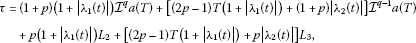(3.3)(3.4)

Theorem 3.1 Assume that

(H1) there exist nonnegative functions $a\left(t\right),b\left(t\right)\in L\left(0,T\right)$ and positive constants ${L}_{i}$ ($i=2,3$) such that

$|f\left(t,x\right)|\le a\left(t\right)+b\left(t\right){|x|}^{\theta },\phantom{\rule{2em}{0ex}}0<\theta <1,\phantom{\rule{2em}{0ex}}|{I}_{k}\left(x\right)|\le {L}_{2},\phantom{\rule{2em}{0ex}}|{I}_{k}^{\ast }\left(x\right)|\le {L}_{3},$

for $t\in J$, $x\in \mathbb{R}$ and $k=1,2,\dots ,p$.

Then the problem (1.1) has at least one solution.

Proof Define a ball $\mathcal{B}=\left\{x\in PC\left(J,\mathbb{R}\right):\parallel x\parallel \le R\right\}$, we just need to show that the operator $\mathfrak{G}:\mathcal{B}\to \mathcal{B}$, as it has already been proved that the operator $\mathfrak{G}:PC\left(J,\mathbb{R}\right)\to PC\left(J,\mathbb{R}\right)$ is completely continuous in the previous lemma. Let us choose $R\ge max\left\{2\tau ,{\left(2\nu \right)}^{\frac{1}{1-\theta }}\right\}$. For any $x\in \mathcal{B}$, by the assumption $\left({H}_{1}\right)$, we have

$\begin{array}{rcl}|\mathfrak{G}x\left(t\right)|& \le & {\int }_{{t}_{k}}^{t}\frac{{\left(t-s\right)}^{q-1}}{\mathrm{\Gamma }\left(q\right)}|a\left(s\right)+b\left(s\right){|x\left(s\right)|}^{\theta }|\phantom{\rule{0.2em}{0ex}}ds\\ +|{\lambda }_{1}\left(t\right)|{\int }_{{t}_{p}}^{T}\frac{{\left(T-s\right)}^{q-1}}{\mathrm{\Gamma }\left(q\right)}|a\left(s\right)+b\left(s\right){|x\left(s\right)|}^{\theta }|\phantom{\rule{0.2em}{0ex}}ds\\ +|{\lambda }_{2}\left(t\right)|{\int }_{{t}_{p}}^{T}\frac{{\left(T-s\right)}^{q-2}}{\mathrm{\Gamma }\left(q-1\right)}|a\left(s\right)+b\left(s\right){|x\left(s\right)|}^{\theta }|\phantom{\rule{0.2em}{0ex}}ds\\ +\sum _{i=1}^{k}\left[{\int }_{{t}_{i-1}}^{{t}_{i}}\frac{{\left({t}_{i}-s\right)}^{q-1}}{\mathrm{\Gamma }\left(q\right)}|a\left(s\right)+b\left(s\right){|x\left(s\right)|}^{\theta }|\phantom{\rule{0.2em}{0ex}}ds+|{I}_{i}\left(x\left({t}_{i}\right)\right)|\right]\\ +\sum _{i=1}^{k-1}\left({t}_{k}-{t}_{i}\right)\left[{\int }_{{t}_{i-1}}^{{t}_{i}}\frac{{\left({t}_{i}-s\right)}^{q-2}}{\mathrm{\Gamma }\left(q-1\right)}|a\left(s\right)+b\left(s\right){|x\left(s\right)|}^{\theta }|\phantom{\rule{0.2em}{0ex}}ds+|{I}_{i}^{\ast }\left(x\left({t}_{i}\right)\right)|\right]\\ +\sum _{i=1}^{k}\left(t-{t}_{k}\right)\left[{\int }_{{t}_{i-1}}^{{t}_{i}}\frac{{\left({t}_{i}-s\right)}^{q-2}}{\mathrm{\Gamma }\left(q-1\right)}|a\left(s\right)+b\left(s\right){|x\left(s\right)|}^{\theta }|\phantom{\rule{0.2em}{0ex}}ds+|{I}_{i}^{\ast }\left(x\left({t}_{i}\right)\right)|\right]\\ +|{\lambda }_{1}\left(t\right)|\sum _{i=1}^{p}\left[{\int }_{{t}_{i-1}}^{{t}_{i}}\frac{{\left({t}_{i}-s\right)}^{q-1}}{\mathrm{\Gamma }\left(q\right)}|a\left(s\right)+b\left(s\right){|x\left(s\right)|}^{\theta }|\phantom{\rule{0.2em}{0ex}}ds+|{I}_{i}\left(x\left({t}_{i}\right)\right)|\right]\\ +|{\lambda }_{1}\left(t\right)|\sum _{i=1}^{p-1}\left({t}_{p}-{t}_{i}\right)\left[{\int }_{{t}_{i-1}}^{{t}_{i}}\frac{{\left({t}_{i}-s\right)}^{q-2}}{\mathrm{\Gamma }\left(q-1\right)}|a\left(s\right)+b\left(s\right){|x\left(s\right)|}^{\theta }|\phantom{\rule{0.2em}{0ex}}ds+|{I}_{i}^{\ast }\left(x\left({t}_{i}\right)\right)|\right]\\ +\sum _{i=1}^{p}\left[\left(T-{t}_{p}\right)|{\lambda }_{1}\left(t\right)|+|{\lambda }_{2}\left(t\right)|\right]\\ ×\left[{\int }_{{t}_{i-1}}^{{t}_{i}}\frac{{\left({t}_{i}-s\right)}^{q-2}}{\mathrm{\Gamma }\left(q-1\right)}|a\left(s\right)+b\left(s\right){|x\left(s\right)|}^{\theta }|\phantom{\rule{0.2em}{0ex}}ds+|{I}_{i}^{\ast }\left(x\left({t}_{i}\right)\right)|\right]\\ \le & \left\{\left(1+p\right)\left(1+|{\lambda }_{1}\left(t\right)|\right){\mathcal{I}}^{q}a\left(T\right)\\ +\left[\left(2p-1\right)T\left(1+|{\lambda }_{1}\left(t\right)|\right)+\left(1+p\right)|{\lambda }_{2}\left(t\right)|\right]{\mathcal{I}}^{q-1}a\left(T\right)\\ +p\left(1+|{\lambda }_{1}\left(t\right)|\right){L}_{2}+\left[\left(2p-1\right)T\left(1+|{\lambda }_{1}\left(t\right)|\right)+p|{\lambda }_{2}\left(t\right)|\right]{L}_{3}\right\}\\ +\left\{\left(1+p\right)\left(1+|{\lambda }_{1}\left(t\right)|\right){\mathcal{I}}^{q}b\left(T\right)\\ +\left[\left(2p-1\right)T\left(1+|{\lambda }_{1}\left(t\right)|\right)+\left(1+p\right)|{\lambda }_{2}\left(t\right)|\right]{\mathcal{I}}^{q-1}b\left(T\right)\right\}{R}^{\theta },\end{array}$
(3.5)

which implies that

$\parallel \mathfrak{G}x\parallel \le \tau +\nu {R}^{\theta }\le \frac{R}{2}+\frac{R}{2}=R,$

where τ and ν are given by (3.3) and (3.4). So, $\mathfrak{G}:\mathcal{B}\to \mathcal{B}$. Thus $\mathfrak{G}:\mathcal{B}\to \mathcal{B}$ is completely continuous. Therefore, by the Schauder fixed point theorem, the operator $\mathfrak{G}$ has at least one fixed point. Consequently, the problem (1.1) has at least one solution in $\mathcal{B}$. □

Theorem 3.2 Assume that

(${H}_{1}^{\prime }$) there exist nonnegative functions $a\left(t\right),b\left(t\right)\in L\left(0,T\right)$ and positive constants ${L}_{i}$ ($i=2,3$) such that

$|f\left(t,x\right)|\le a\left(t\right)+b\left(t\right){|x|}^{\kappa },\phantom{\rule{2em}{0ex}}\kappa >1,\phantom{\rule{2em}{0ex}}|{I}_{k}\left(x\right)|\le {L}_{2},\phantom{\rule{2em}{0ex}}|{I}_{k}^{\ast }\left(x\right)|\le {L}_{3},$

for $t\in J$, $x\in \mathbb{R}$ and $k=1,2,\dots ,p$.

Then the problem (1.1) has at least one solution.

Proof The proof is similar to that of Theorem 3.1, so we omit it. □

Theorem 3.3 ()

Let E be a Banach space. Assume that $\mathfrak{G}:E\to E$ is a completely continuous operator and the set $V=\left\{x\in E|x=\mu Tx,0<\mu <1\right\}$ is bounded. Then $\mathfrak{G}$ has a fixed point in E.

Theorem 3.4 If ${sup}_{t\in J}\nu <1$. In addition, assume that

(${H}_{1}^{″}$) there exist nonnegative functions $a\left(t\right),b\left(t\right)\in L\left(0,T\right)$ and positive constants ${L}_{i}$ ($i=2,3$) such that

$|f\left(t,x\right)|\le a\left(t\right)+b\left(t\right)|x|,\phantom{\rule{2em}{0ex}}|{I}_{k}\left(x\right)|\le {L}_{2},\phantom{\rule{2em}{0ex}}|{I}_{k}^{\ast }\left(x\right)|\le {L}_{3},$

for $t\in J$, $x\in \mathbb{R}$ and $k=1,2,\dots ,p$.

Then the problem (1.1) has at least one solution.

Proof Let us consider the set

$V=\left\{x\in PC\left(J,\mathbb{R}\right)|x=\mu \mathfrak{G}x,0<\mu <1\right\},$

where the operator $\mathfrak{G}:PC\left(J,\mathbb{R}\right)\to PC\left(J,\mathbb{R}\right)$ is defined by (3.1). We just need to show that the set V is bounded as it has already been proved that the operator $\mathfrak{G}$ is completely continuous in the previous lemma. Let $x\in V$, then $x=\mu \mathfrak{G}x$, $0<\mu <1$. For any $t\in J$, we have

$\begin{array}{rcl}|x\left(t\right)|& =& \mu |\mathfrak{G}x\left(t\right)|\\ \le & {\int }_{{t}_{k}}^{t}\frac{{\left(t-s\right)}^{q-1}}{\mathrm{\Gamma }\left(q\right)}|f\left(s,x\left(s\right)\right)|\phantom{\rule{0.2em}{0ex}}ds+|{\lambda }_{1}\left(t\right)|{\int }_{{t}_{p}}^{T}\frac{{\left(T-s\right)}^{q-1}}{\mathrm{\Gamma }\left(q\right)}|f\left(s,x\left(s\right)\right)|\phantom{\rule{0.2em}{0ex}}ds\\ +|{\lambda }_{2}\left(t\right)|{\int }_{{t}_{p}}^{T}\frac{{\left(T-s\right)}^{q-2}}{\mathrm{\Gamma }\left(q-1\right)}|f\left(s,x\left(s\right)\right)|\phantom{\rule{0.2em}{0ex}}ds\\ +\sum _{i=1}^{k}\left[{\int }_{{t}_{i-1}}^{{t}_{i}}\frac{{\left({t}_{i}-s\right)}^{q-1}}{\mathrm{\Gamma }\left(q\right)}|f\left(s,x\left(s\right)\right)|\phantom{\rule{0.2em}{0ex}}ds+|{I}_{i}\left(x\left({t}_{i}\right)\right)|\right]\\ +\sum _{i=1}^{k-1}\left({t}_{k}-{t}_{i}\right)\left[{\int }_{{t}_{i-1}}^{{t}_{i}}\frac{{\left({t}_{i}-s\right)}^{q-2}}{\mathrm{\Gamma }\left(q-1\right)}|f\left(s,x\left(s\right)\right)|\phantom{\rule{0.2em}{0ex}}ds+|{I}_{i}^{\ast }\left(x\left({t}_{i}\right)\right)|\right]\\ +\sum _{i=1}^{k}\left(t-{t}_{k}\right)\left[{\int }_{{t}_{i-1}}^{{t}_{i}}\frac{{\left({t}_{i}-s\right)}^{q-2}}{\mathrm{\Gamma }\left(q-1\right)}|f\left(s,x\left(s\right)\right)|\phantom{\rule{0.2em}{0ex}}ds+|{I}_{i}^{\ast }\left(x\left({t}_{i}\right)\right)|\right]\\ +|{\lambda }_{1}\left(t\right)|\sum _{i=1}^{p}\left[{\int }_{{t}_{i-1}}^{{t}_{i}}\frac{{\left({t}_{i}-s\right)}^{q-1}}{\mathrm{\Gamma }\left(q\right)}|f\left(s,x\left(s\right)\right)|\phantom{\rule{0.2em}{0ex}}ds+|{I}_{i}\left(x\left({t}_{i}\right)\right)|\right]\\ +|{\lambda }_{1}\left(t\right)|\sum _{i=1}^{p-1}\left({t}_{p}-{t}_{i}\right)\left[{\int }_{{t}_{i-1}}^{{t}_{i}}\frac{{\left({t}_{i}-s\right)}^{q-2}}{\mathrm{\Gamma }\left(q-1\right)}|f\left(s,x\left(s\right)\right)|\phantom{\rule{0.2em}{0ex}}ds+|{I}_{i}^{\ast }\left(x\left({t}_{i}\right)\right)|\right]\\ +\sum _{i=1}^{p}\left[\left(T-{t}_{p}\right)|{\lambda }_{1}\left(t\right)|+|{\lambda }_{2}\left(t\right)|\right]\left[{\int }_{{t}_{i-1}}^{{t}_{i}}\frac{{\left({t}_{i}-s\right)}^{q-2}}{\mathrm{\Gamma }\left(q-1\right)}|f\left(s,x\left(s\right)\right)|\phantom{\rule{0.2em}{0ex}}ds+|{I}_{i}^{\ast }\left(x\left({t}_{i}\right)\right)|\right]\\ \le & \tau +\nu \parallel x\parallel ,\end{array}$

which implies that $\parallel x\parallel$ is bounded for any $t\in J$. So, the set V is bounded. Thus, by the conclusion of Theorem 3.3, the operator $\mathfrak{G}$ has at least one fixed point, which implies that (1.1) has at least one solution. □

Corollary 3.1 Assume that functions f, ${I}_{k}$, ${I}_{k}^{\ast }$ ($k=1,2,\dots$) are bounded. Then the nonlinear problem (1.1) has at least one solution.

Theorem 3.5 Assume that

(${H}_{2}$) there exist a nonnegative function ${K}_{1}\left(t\right)\in L\left(0,T\right)$ and positive constants ${K}_{i}$ ($i=2,3$) such that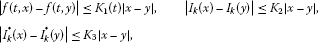for $t\in J$, $x,y\in \mathbb{R}$ and $k=1,2,\dots ,p$.

Then the problem (1.1) has a unique solution if

$\begin{array}{rcl}\mathcal{H}& =& \underset{t\in J}{max}\left\{\left(1+p\right)\left(1+|{\lambda }_{1}\left(t\right)|\right){\mathcal{I}}^{q}{K}_{1}\left(T\right)\\ +\left[\left(2p-1\right)T\left(1+|{\lambda }_{1}\left(t\right)|\right)+\left(1+p\right)|{\lambda }_{2}\left(t\right)|\right]{\mathcal{I}}^{q-1}{K}_{1}\left(T\right)\\ +p\left(1+|{\lambda }_{1}\left(t\right)|\right){K}_{2}+\left[\left(2p-1\right)T\left(1+|{\lambda }_{1}\left(t\right)|\right)+p|{\lambda }_{2}\left(t\right)|\right]{K}_{3}\right\}<1.\end{array}$
(3.6)

Proof For $x,y\in PC\left(J,\mathbb{R}\right)$, we can get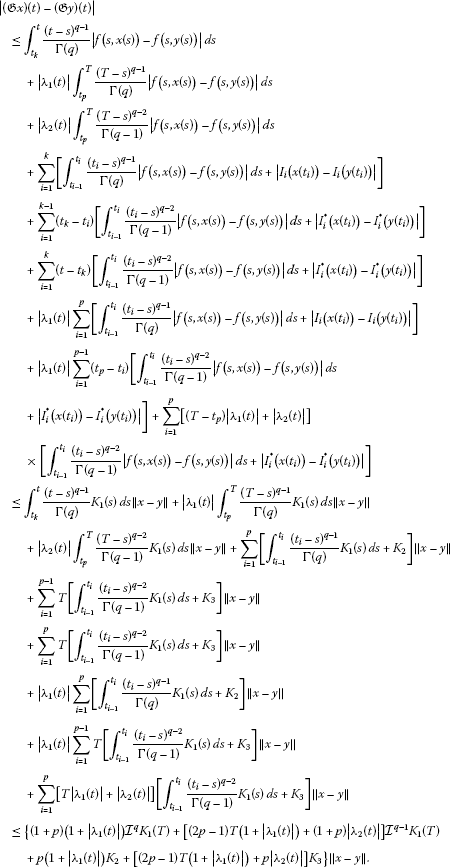Consequently, we have $\parallel \mathfrak{G}x-\mathfrak{G}y\parallel \le \mathcal{H}\parallel x-y\parallel$, where $\mathcal{H}$ is given by (3.6). As $\mathcal{H}<1$, the conclusion of the theorem follows by the contraction mapping principle. This completes the proof. □

## 4 Examples

Example 4.1 Consider the following impulsive fractional boundary value problem with closed boundary conditions

$\left\{\begin{array}{c}{}^{C}D^{q}x\left(t\right)=\frac{{e}^{-{sin}^{2}x\left(t\right)}\left[2+sin2t+{|x\left(t\right)|}^{\rho }ln\left(1+2{cos}^{2}t\right)\right]}{2+cosx\left(t\right)},\phantom{\rule{1em}{0ex}}0
(4.1)

where $1, $0<\rho <1$ and $p=1$.

In this case, $a\left(t\right)=2+sin2t$, $b\left(t\right)=ln\left(1+2{cos}^{2}t\right)$, ${L}_{2}=1$, ${L}_{3}=5$, and the conditions of Theorem 3.1 can readily be verified. Thus, by the conclusion of Theorem 3.1, the problem (4.1) has at least one solution.

## References

1. Lakshmikantham V, Bainov DD, Simeonov PS: Theory of Impulsive Differential Equations. World Scientific, Singapore; 1989.

2. Samoilenko AM, Perestyuk NA: Impulsive Differential Equations. World Scientific, Singapore; 1995.

3. Krogh B, Lynch N (Eds): Lecture Notes in Computer Science 1790 In Hybrid Systems: Computation and Control. Springer, New York; 2000.

4. Vaandrager F, Schuppen J (Eds): Lecture Notes in Computer Science 1569 In Hybrid Systems: Computation and Control. Springer, New York; 1999.

5. Engell S, Frehse G, Schnieder E Lecture Notes in Control and Information Sciences. In Modelling, Analysis and Design of Hybrid Systems. Springer, Heidelberg; 2002.

6. Antsaklis PJ, Nerode A: Hybrid control systems: an introductory discussion to the special issue. IEEE Trans. Autom. Control 1998, 43: 457–460. 10.1109/TAC.1998.664148

7. Kilbas AA, Srivastava HM, Trujillo JJ North-Holland Mathematics Studies 204. In Theory and Applications of Fractional Differential Equations. Elsevier, Amsterdam; 2006.

8. Sabatier J, Agrawal OP, Machado JAT (Eds): Advances in Fractional Calculus: Theoretical Developments and Applications in Physics and Engineering. Springer, Dordrecht; 2007.

9. Lakshmikantham V, Leela S, Devi JV: Theory of Fractional Dynamic Systems. Cambridge Academic Publishers, Cambridge; 2009.

10. Ahmad B, Nieto JJ: Existence results for a coupled system of nonlinear fractional differential equations with three-point boundary conditions. Comput. Math. Appl. 2009, 58: 1838–1843. 10.1016/j.camwa.2009.07.091

11. Wei Z, Li Q, Che J: Initial value problems for fractional differential equations involving Riemann-Liouville sequential fractional derivative. J. Math. Anal. Appl. 2010, 367: 260–272. 10.1016/j.jmaa.2010.01.023

12. Zhang SQ: Positive solutions to singular boundary value problem for nonlinear fractional differential equation. Comput. Math. Appl. 2010, 59: 1300–1309. 10.1016/j.camwa.2009.06.034

13. Zhou Y, Jiao F: Nonlocal Cauchy problem for fractional evolution equations. Nonlinear Anal., Real World Appl. 2010, 11: 4465–4475. 10.1016/j.nonrwa.2010.05.029

14. Ahmad B, Nieto JJ, Pimentel J: Some boundary value problems of fractional differential equations and inclusions. Comput. Math. Appl. 2011, 62: 1238–1250. 10.1016/j.camwa.2011.02.035

15. Wang G, Ntouyas SK, Zhang L: Positive solutions of the three-point boundary value problem for fractional-order differential equations with an advanced argument. Adv. Differ. Equ. 2011., 2011: Article ID 2

16. Ford NJ, Morgado ML: Fractional boundary value problems: analysis and numerical methods. Fract. Calc. Appl. Anal. 2011, 14: 554–567.

17. Aghajani A, Jalilian Y, Trujillo JJ: On the existence of solutions of fractional integro-differential equations. Fract. Calc. Appl. Anal. 2012, 15: 44–69.

18. Ahmad B, Nieto JJ: Anti-periodic fractional boundary value problem with nonlinear term depending on lower order derivative. Fract. Calc. Appl. Anal. 2012, 15: 451–462.

19. Baleanu D, Diethelm K, Scalas E, Trujillo JJ Series on Complexity, Nonlinearity and Chaos. In Fractional Calculus Models and Numerical Methods. World Scientific, Boston; 2012.

20. Ahmad B, Nieto JJ, Alsaedi A, El-Shahed M: A study of nonlinear Langevin equation involving two fractional orders in different intervals. Nonlinear Anal., Real World Appl. 2012, 13: 599–606. 10.1016/j.nonrwa.2011.07.052

21. Wang G, Agarwal RP, Cabada A: Existence results and the monotone iterative technique for systems of nonlinear fractional differential equations. Appl. Math. Lett. 2012, 25: 1019–1024. 10.1016/j.aml.2011.09.078

22. Ahmad B, Ntouyas SK: Nonlinear fractional differential equations and inclusions of arbitrary order and multi-strip boundary conditions. Electron. J. Differ. Equ. 2012., 2012: Article ID 98

23. Chen L, Sun J: Nonlinear boundary value problem of first order impulsive functional differential equations. J. Math. Anal. Appl. 2006, 318(2):726–741. 10.1016/j.jmaa.2005.08.012

24. Luo Z, Nieto JJ: New results for the periodic boundary value problem for impulsive integro-differential equations. Nonlinear Anal. 2009, 70(6):2248–2260. 10.1016/j.na.2008.03.004

25. Li C, Sun J, Sun R: Stability analysis of a class of stochastic differential delay equations with nonlinear impulsive effects. J. Franklin Inst. 2010, 347: 1186–1198. 10.1016/j.jfranklin.2010.04.017

26. Tian Y, Bai Z: Existence results for the three-point impulsive boundary value problem involving fractional differential equations. Comput. Math. Appl. 2010, 59: 2601–2609. 10.1016/j.camwa.2010.01.028

27. Wang G, Ahmad B, Zhang L: Impulsive anti-periodic boundary value problem for nonlinear differential equations of fractional order. Nonlinear Anal. 2011, 74: 792–804. 10.1016/j.na.2010.09.030

28. Ahmad B, Wang G: A study of an impulsive four-point nonlocal boundary value problem of nonlinear fractional differential equations. Comput. Math. Appl. 2011, 62: 1341–1349. 10.1016/j.camwa.2011.04.033

29. Bai C: Impulsive periodic boundary value problems for fractional differential equation involving Riemann-Liouville sequential fractional derivative. J. Math. Anal. Appl. 2011, 384: 211–231. 10.1016/j.jmaa.2011.05.082

30. Ahmad B, Nieto JJ: Existence of solutions for impulsive anti-periodic boundary value problems of fractional order. Taiwan. J. Math. 2011, 15: 981–993.

31. Mu J, Li Y: Monotone iterative technique for impulsive fractional evolution equations. J. Inequal. Appl. 2011., 2011: Article ID 125

32. Sun JX: Nonlinear Functional Analysis and Its Application. Science Press, Beijing; 2008.

## Acknowledgements

We would like to express our gratitude to the anonymous reviewers and editors for their valuable comments and suggestions that led to the improvement of the original manuscript. The research of B. Ahmad was partially supported by Deanship of Scientific Research (DSR), King Abdulaziz University, Jeddah, Saudi Arabia. The research of Guotao Wang and Lihong Zhang was supported by the Natural Science Foundation for Young Scientists of Shanxi Province (2012021002-3), China.

## Author information

Authors

### Corresponding author

Correspondence to Lihong Zhang.

### Competing interests

The authors declare that they have no competing interests.

## Rights and permissions

Reprints and Permissions

Wang, G., Ahmad, B. & Zhang, L. Existence results for nonlinear fractional differential equations with closed boundary conditions and impulses. Adv Differ Equ 2012, 169 (2012). https://doi.org/10.1186/1687-1847-2012-169

• Accepted:

• Published:

• DOI: https://doi.org/10.1186/1687-1847-2012-169

### Keywords

• nonlinear fractional differential equations
• impulse
• closed boundary conditions
• fixed point theorem